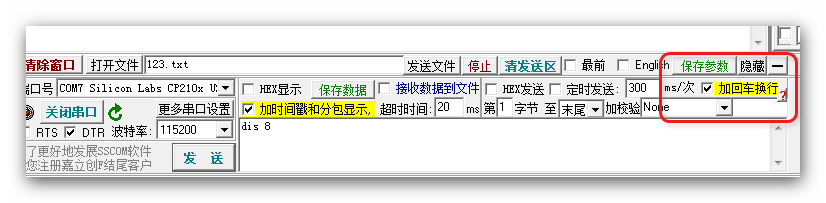2016-01-03 11:12:13 aorangezzz 阅读数 1334
• ###### 《C语言基础》 之 第9章 字符串

课程内容：字符数组1、字符串越位的问题、指针变量的空间大小、字符串常量、字符串传参、printf格式化输出字符串、字符串的非格式化输出函数、字符串输入函数、strlen函数、strcpy函数和strncpy函数、strcmp函数和strncmp函数、strcat函数和strncat函数。

3452 人正在学习 去看看 张先凤
```/***********************************************************************************
*	标题:                  RS232串口通信试验                                        *
*	功能：串口接收数据后打印，按回车键处理命令，通过数组储存命令				    *
*	      按 退格键 会准确地删除一位数据并清除串口的显示，尝试使用串口命令来清屏    *
*************************************************************************************/

#include <REG52.H>

sbit BEEP = P1^4;
sbit JDQ1 = P1^1;
sbit JDQ2 = P1^2;

bit Flag;
bit Flag_comm;
unsigned char ReData,SenData;	//接收数据缓存，发送数据缓存	，不指定大小会waring，应该是怕溢出

static unsigned char coun = 0;

void serial_print(char *str){
unsigned char i = 0;
while(str[i] != '\0')
{
SBUF = str[i];
while(!TI);
TI = 0;
i++;
}
}

//串口初始化，初始化函数需要改进，修改为自动计算配置
void serial_init(){
SCON = 0x50;      //REN=1允许串行接受状态，串口工作模式1
TMOD|= 0x20;      //定时器工作方式2
PCON|= 0x80;
TH1  = 0xFD;     //baud*2  /* reload value 19200、数据位8、停止位1。效验位无 (11.0592)
//	TH1 = 0xF3;					// //baud*2  /*  波特率4800、数据位8、停止位1。效验位无 (12M)
TL1 = 0xF3;
TR1  = 1;
}

//主函数，串口接收完整指令后解析执行命令
void main (void) {
serial_init();    //串口初始化要加入配置
ES   = 1;        //开串口中断
EA   = 1;        // 开总中断

serial_print("\n\rserial_init,waiting for input:\n\r");
serial_print("common  input:");
while(1)
{
if(Flag_comm == 1){
serial_print("\n\r");
serial_print("\n\r");
//这里将传入参数进行命令解析
serial_print("excuseing !!!!!!!!!!!!\n\r");
serial_print("common  input:");
coun = 0;   //打印后清零串口计数
Flag_comm = 0;	//处理命令后清除标志位
}
}
}

/****************************************************
串口中断程序
******************************************************/
void serial_interrupt (void) interrupt 4 using 1
{
if(RI == 1)        //RI接受中断标志
{
RI = 0;		    //清除RI接受中断标志
Flag=1;		    //接收到数据
*ReData = SBUF;  //SUBF接受/发送缓冲器
*SenData = *ReData;
if( *ReData != 0x0d ){	   //等待接收回车
if( *ReData == 0x08 ){	 //退格键
serial_print("\b ");               //覆盖掉原来的值
}else{
}
}else{
Flag_comm = 1;					 //接收命令完成，解析命令
}
}
if(Flag == 1){		 //接收到字符就打印，避免存在输入过快无法显示
serial_print(SenData);
Flag = 0;
}
}
//0x0d = \r 0x0a = \n	0x08 = \b```

2016-04-15 17:02:17 CB_869145753_hp 阅读数 30426
• ###### 《C语言基础》 之 第9章 字符串

课程内容：字符数组1、字符串越位的问题、指针变量的空间大小、字符串常量、字符串传参、printf格式化输出字符串、字符串的非格式化输出函数、字符串输入函数、strlen函数、strcpy函数和strncpy函数、strcmp函数和strncmp函数、strcat函数和strncat函数。

3452 人正在学习 去看看 张先凤

void serial_port_one_init()
{                       //根据自己单片机设置；

//22.1184M   波特率： 115200
SCON = 0x50;
BRT = 0xFA;
AUXR |= 0x04;
AUXR |= 0x01;
AUXR |= 0x10;
ES      =   1;
EA      =   1;
}

#define Data_SIZE 10 //数据长度   9位数据 +  /r/n  -  /n =10位

char RevBuf[Data_SIZE];      //数据接收缓冲区
char temp[Data_SIZE];        //防数据抵消缓冲区
unsigned char flished_flag=0; //数据接收符合要求标志
int data_count=0;    //数据长度
int temp_length;    //数据长度
int data_flished_count = 0;  //
char data_flished;           //

{
uchar temp;
if(RI==1)
{
RI=0;
temp=SBUF;
//        senduart(temp);  //用来测试过数据接收是否正确

if(temp!='\n') //判断是否接收到结束符
{
RevBuf[data_count]=temp;// 否，就存到RevBuf【】数组中
data_count++;
}
else
{
temp_length=data_count;//是，记录其数据长度
data_count=0;
}

}

}

void main(void)

{

serial_port_one_init();  //串口初始化

while(1)

{

if(Data_SIZE == temp_length)   //判断数据长度是否满足我们的要求。
{
for(i=0;i<Wifi_Data_SIZE;i++)
{
temp[i]=RevBuf[i];             // 同时我们将temp【】作为缓冲区，防止数据被冲到
}
flished_flag=1;          //数据接收成功标志
}

if(1==flished_flag)  //     数据接收完整成功
{

wifi_flished_flag=0;  //

//

//你想要实现的功能

/*

switch(temp) //我常把数据第一位或前几位作为指令，后几位作为数据，你也可以把整个发送的数据就作为指令。

{

case 'A' :

//具体操作

break;

}

*/

}

}

while(1);

}2014-03-31 21:50:51 u014030821 阅读数 21647
• ###### 《C语言基础》 之 第9章 字符串

课程内容：字符数组1、字符串越位的问题、指针变量的空间大小、字符串常量、字符串传参、printf格式化输出字符串、字符串的非格式化输出函数、字符串输入函数、strlen函数、strcpy函数和strncpy函数、strcmp函数和strncmp函数、strcat函数和strncat函数。

3452 人正在学习 去看看 张先凤

```#include <reg52.h>
#define uint unsigned int
#define uchar unsigned char
//定义接收 字符
uchar Buffer;

//串口初始化函数
void   URATinit( )
{
TMOD=0x20;
SCON=0x50;
EA=1;
ES=1;
TR1=1;
TH1=0xfd;
TL1=0xfd;
}
//中断函数
{
if(RI)
{
Buffer=SBUF;
RI=0;
}
SBUF=Buffer;
while(!TI);
TI=0;
}
//主函数
void  main()
{
URATinit( );
}```（1）

```#include <reg52.h>
#define uint unsigned int
#define uchar unsigned char
//定义接收 数组
uchar Buffer={0};
uchar i=0,j=0;

//串口初始化函数
void   URATinit( )
{
TMOD=0x20;
SCON=0x50;
EA=1;
ES=1;
TR1=1;
TH1=0xfd;
TL1=0xfd;
}
//中断函数
{
if(RI)
{
Buffer[i]=SBUF;
RI=0;
}
SBUF=Buffer[i];
while(!TI)	;
TI=0;
i++;
if(i>=5){
i=0;
}
}
//主函数
void  main()
{
URATinit( );
}		 ```（2）

```#include <reg52.h>
#define uint unsigned int
#define uchar unsigned char
//定义接收 数组
uchar Buffer;
uchar i=0,flag;

//延时函数
delay(uint  ms)
{
uchar i;
while(ms--)
for(i=0;i<123;i++);
}
//串口初始化函数
void   URATinit( )
{
TMOD=0x20;
SCON=0x50;
EA=1;
ES=1;
TR1=1;
TH1=0xfd;
TL1=0xfd;
}
//中断函数
{
if(RI)
{
Buffer[i++]=SBUF;
RI=0;
if(i>=5){
i=0;
}
flag=1;
}
}
//主函数
void  main()
{
uchar k=0;
for(k;k<5;k++){
Buffer[k]=0;
}
URATinit( );
while(1){
if(flag) {
uchar j=0;
for(j;j<5;j++){
SBUF=Buffer[j];
while(!TI)	;
TI=0;
delay(50);
}
flag=0;
}
}
}
``````#include<reg52.h>
#define uchar unsigned char
#define uint unsigned int
uchar num;

sbit dula=P2^6;		//申明U1锁存器的锁存端
sbit wela=P2^7;		//申明U2锁存器的锁存端

uchar code table[]={
0x3f,0x06,0x5b,0x4f,
0x66,0x6d,0x7d,0x07,
0x7f,0x6f,0x77,0x7c,
0x39,0x5e,0x79,0x71};

void delay(uint xms)
{
uint i,j;
for(i=xms;i>0;i--)		      //i=xms即延时约xms毫秒
for(j=110;j>0;j--);
}

void display(uint value)  //显示子函数
{
uchar wan,qian,bai,shi,ge;   //定义万千百十个位
wan=value/10000;
qian=value%10000/1000;
bai=value%1000/100;
shi=value%100/10;
ge=value%10;

dula=1;
P0=table[wan];
dula=0;
P0=0xff;
wela=1;
P0=0xfe;
wela=0;
delay(2);

dula=1;
P0=table[qian];
dula=0;
P0=0xff;
wela=1;
P0=0xfd;
wela=0;
delay(2);

dula=1;
P0=table[bai];
dula=0;
P0=0xff;
wela=1;
P0=0xfb;
wela=0;
delay(2);

dula=1;
P0=table[shi];
dula=0;
P0=0xff;
wela=1;
P0=0xf7;
wela=0;
delay(2);

dula=1;
P0=table[ge];
dula=0;
P0=0xff;
wela=1;
P0=0xef;
wela=0;
delay(2);
}

void init()         //初始化函数
{
TMOD=0x20;      //设置定时器1工作方式
TH1=0xfd;
TL1=0xfd;
TR1=1;
SM0=0;
SM1=1;
REN=1;
EA=1;
ES=1;
}

void main()
{
init();
while(1)
{
display(num);
}

}

void ser() interrupt 4     //串口中断函数
{
if(RI){
num=SBUF;
RI=0;
}              //置RI为0以便接收下一个数据
SBUF=num;
while(!TI);
TI=0;
}```2019-07-19 19:52:57 gajicat 阅读数 114
• ###### 《C语言基础》 之 第9章 字符串

课程内容：字符数组1、字符串越位的问题、指针变量的空间大小、字符串常量、字符串传参、printf格式化输出字符串、字符串的非格式化输出函数、字符串输入函数、strlen函数、strcpy函数和strncpy函数、strcmp函数和strncmp函数、strcat函数和strncat函数。

3452 人正在学习 去看看 张先凤

``````void timer0() interrupt TIMER0_VECTOR

{
TR0=0;
if(recv_index>0){

if(recv_index<BUFLEN-1){
recv_str[recv_index]=last_buf;
recv_index++;

}
if(recv_index<BUFLEN){
recv_str[recv_index]=0;
strcpy(cmd_str,recv_str);
get_line = 1;

}else{
recv_str[BUFLEN-1]=0;
}
}
last_buf=0;
recv_index=0;
}

void UART1_int (void) interrupt UART1_VECTOR
{
//u8 last_index;
TR0 = 0;
if(RI)
{
RI = 0;
recv_buf = SBUF;
if(recv_buf == '\n' && last_buf=='\r'){
if(recv_index < BUFLEN){
recv_str[recv_index]=0;
strcpy(cmd_str,recv_str);
get_line = 1;
}else{
recv_str[BUFLEN-1]=0;
}
last_buf=0;
recv_index = 0;

}else{
if(recv_index < BUFLEN){
if(last_buf != 0){
recv_str[recv_index]=last_buf;
recv_index++;
}
}
last_buf=recv_buf;
setTimer0();
TR0 = 1;
}
COM1.B_RX_OK = 0;
}

if(TI)
{
TI = 0;
COM1.B_TX_busy = 0;
}
}

``````2019-12-06 14:57:14 xiaoeleis 阅读数 33
• ###### 《C语言基础》 之 第9章 字符串

课程内容：字符数组1、字符串越位的问题、指针变量的空间大小、字符串常量、字符串传参、printf格式化输出字符串、字符串的非格式化输出函数、字符串输入函数、strlen函数、strcpy函数和strncpy函数、strcmp函数和strncmp函数、strcat函数和strncat函数。

3452 人正在学习 去看看 张先凤``````
bit rcvFlg;

UINT16 recvCnt=0;

static UINT8 state=0;

#define UARTRCBUFSIZE  128
UINT8  UART_BUFFER[UARTRCBUFSIZE]；

void main()

{

InitialUART0_Timer3(115200);
set_ES;           //enable UART interrupt 启用串口中断
set_EA;           //enable global interrupt 启用全局中断

while(1)
{

if (rcvFlg)
{

Uart0_SendString(UART_BUFFER);

set_ES;            //enable UART interrupt
SCON = 0x50;      //UART0 Mode1,REN=1,TI=1
rcvFlg= 0;

}

}

}

void serial_IT(void) interrupt 4
{

if (RI)
{
UART_BUFFER[recvCnt++] = SBUF;                                  /* if reception occur */
clr_RI;       /* clear reception flag for next reception */
if((SBUF == '\0')||(SBUF == '\n'))
{
UART_BUFFER[recvCnt]='\0';
state = 1;
}

}
if(TI)
{
clr_TI;                             /* if emission occur */
}

if(state)
{
state = 0;
UART_BUFFER[recvCnt]='\0';
clr_ES;
SCON=0x40;
rcvFlg =1;
recvCnt = 0;
}

}

``````